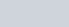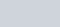If a number x is chosen from the numbers 1, 2, 3,
Question:

If a number x is chosen from the numbers 1, 2, 3, and a number y is selected from the numbers 1, 4, 9. Then, P(xy < 9)

(a) $\frac{7}{9}$

(b) $\frac{5}{9}$

(c) $\frac{2}{3}$

(d) $\frac{1}{9}$

Solution:

GIVEN: x is chosen from the numbers 1, 2, 3 and y is chosen from the numbers 1, 4, 9

TO FIND: Probability of gettingWe will make multiplication table for and y such that$x y=\mid \times 1=1$

$=1 \times 4=4$

$=1 \times 9=9$

$=2 \times 1=2$

$=2 \times 4=8$

$=2 \times 9=18$

$=3 \times 1=3$

$=3 \times 4=12$

$=3 \times 9=27$

So the numbers such that $(x y)<9$ is 5

We know that PROBABILITY $=\frac{\text { Number of favour able event }}{\text { Total number of event }}$

Hence $P(x y<9)=\frac{5}{9}$

Hence the correct option is $(b)$